# EE GATE 2013 - Online Test

Q1. Given a vector field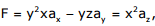the line integral ∫ F.dl evaluated along a segment on the x-axis from x=1 to x=2 is
Explaination / Solution:
No Explaination.

Q2. Statement: You can always give me a ring whenever you need. Which one of the following is the best inference from the above statement?
Explaination / Solution:
No Explaination.

Q3. The curl of the gradient of the scalar field defined by V = 2x2y + 3y2z + 4z2x is
Explaination / Solution:
No Explaination.

Q4. Statement: There were different streams of freedom movements in colonial India carried out by the moderates, liberals, radicals, socialists, and so on. Which one of the following is the best inference from the above statement?
Explaination / Solution:
No Explaination.

Q5. The flux density at a point in space is given by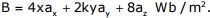The value of constant k must be equal to
Explaination / Solution:
No Explaination.

Q6. In the circuit shown below what is the output voltage out (Vout) in Volts if a silicon transistor Q and an ideal op-amp are used?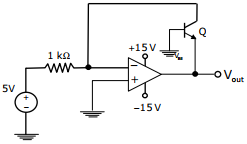Explaination / Solution:
No Explaination.

Q7. A dielectric slab with 500mm x 500mm cross-section is 0.4m long. The slab is subjected to a uniform electric field of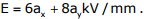. The relative permittivity of the dielectric material is equal to 2. The value of constant ε0 is 8.85 × 10-12 F/m. The energy stored in the dielectric in Joules is
Explaination / Solution:
No Explaination.

Q8. Complete the sentence: Dare ____________ mistakes.
Explaination / Solution:
No Explaination.

Q9. In the circuit shown below the op-amps are ideal. Then Vout in Volts is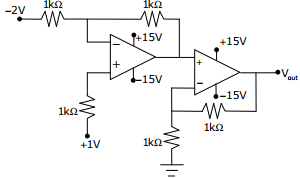Explaination / Solution:
No Explaination.

Q10. Choose the grammatically CORRECT sentence:
Explaination / Solution:
No Explaination.